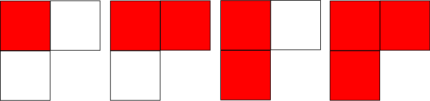### Problem Statement

A Ferrers diagram of the partition of positive number n = a1 + a2 + ... + ak, for a list a1, a2, ..., ak of k positive integers with a1 ≥ a2 ≥ ... ≥ ak is an arrangement of n boxes in k rows, such that the boxes are left-justified, the first row is of length a1, the second row is of length a2, and so on, with the kth row of length ak. Let's call a FIELD diagram of order fieldOrder a Ferrers diagram with a1fieldOrder, a2fieldOrder - 1, ..., akfieldOrder - k + 1, so a FIELD diagram can have a number of rows which is less than or equal to fieldOrder. Your method will be given fieldOrder, it should return the total number of FIELD diagrams of order fieldOrder.

### Definition

 Class: FIELDDiagrams Method: countDiagrams Parameters: int Returns: long Method signature: long countDiagrams(int fieldOrder) (be sure your method is public)

### Constraints

-fieldOrder will be between 1 and 30, inclusive

### Examples

0)

 `2`
`Returns: 4`
 There are four possible FIELD diagrams for fieldOrder equal to 2, corresponding to partitions: (1), (2), (1, 1), (2,1). They are shown in the picture below. There white stands for unused space in a row and red for boxes, corresponding to FIELD diagrams.1)

 `3`
`Returns: 13`
2)

 `5`
`Returns: 131`

#### Problem url:

http://www.topcoder.com/stat?c=problem_statement&pm=8776

#### Problem stats url:

http://www.topcoder.com/tc?module=ProblemDetail&rd=12173&pm=8776

griffon

#### Testers:

PabloGilberto , Olexiy , gawry

Simple Math